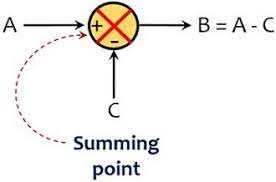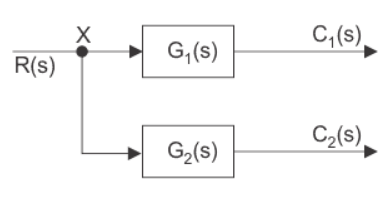### Summing Point

Summing Point:
Summing Point is a point in block diagram representation which outputs the algebraic sum of the inputs. It can have multiple inputs and a single output. The nature of the operation depends on the polarity of the input.Take-off Point:
The take-off point is a point where the input has more than one path to propagate i.e the point from which the same signal can be passed through more than one branch.Block Diagram Representation of Electrical Systems

Any Electrical system can be simplified using block diagram representation just by following the below steps:

• Convert the time domain electrical circuit into an s-domain electrical circuit by using Laplace transform.

• Deduce the equations for the current passing through all series branch elements and voltage across all shunt branches.

• Draw the individual block diagrams for all the above equations.

• Combine all the individual block diagrams to get the overall block diagram representation of the electrical system.